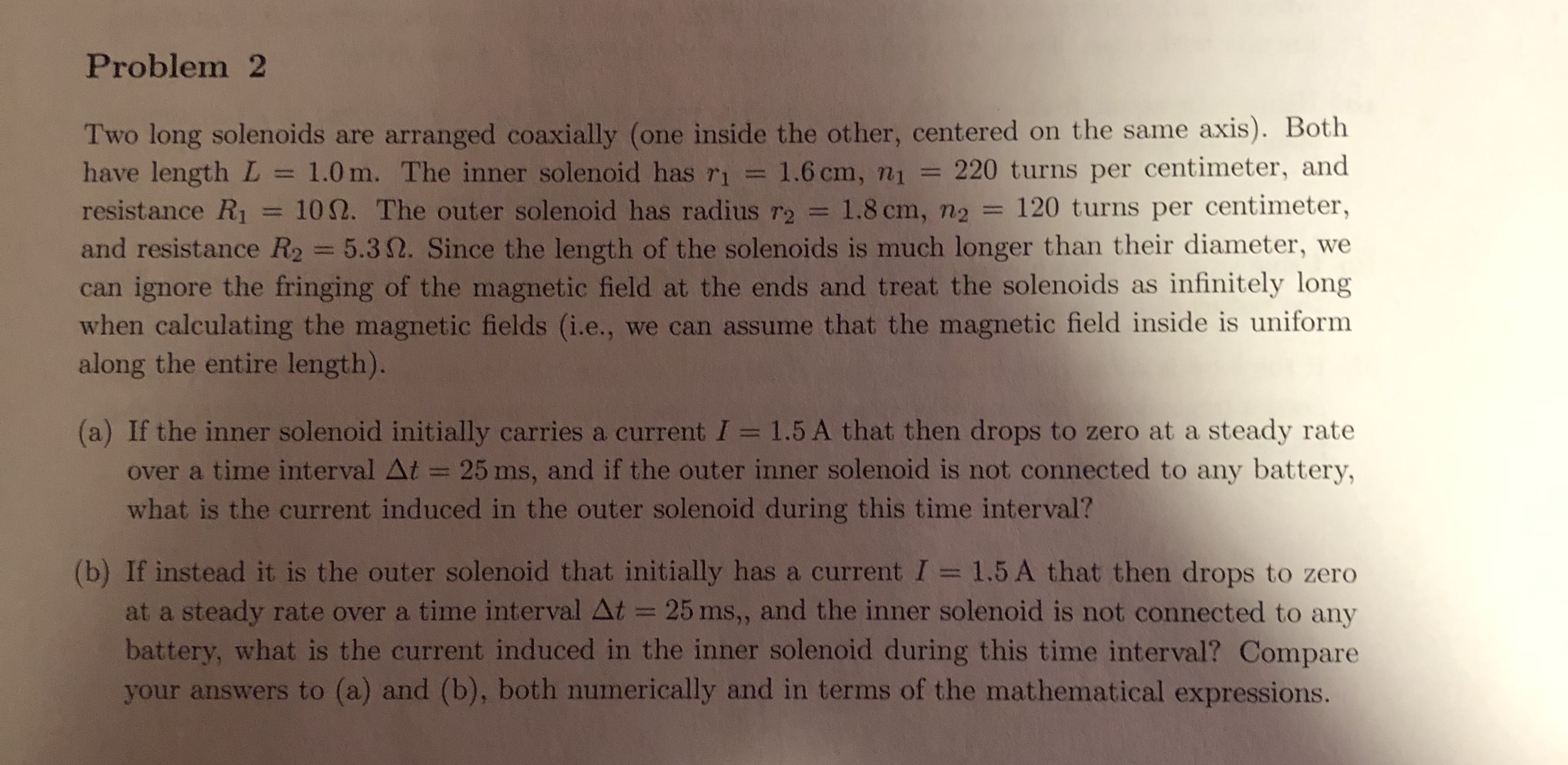# Problem2Two long solenoids are arranged coaxially (one inside the other, centered on the same axis). Bothhave length L 1.0m. The inner solenoid has r1 1.6 cm, n1 220 turns per centimeter, andresistance Ri = 10Ω. The outer solenoid has radius r2 = 1.8 cm, n2 = 120 turns per centimeter,and resistance R2 5.3 Ω. Since the length of the solenoids is much longer than their diameter, wecan ignore the fringing of the magnetic field at the ends and treat the solenoids as infinitely longwhen calculating the magnetic fields (i.e., we can assume that the magnetic field inside is uniformalong the entire length).(a) If the inner solenoid initially carries a current I 1.5 A that then drops to zero at a steady rateover a time interval Δt-: 25 ms, and if the outer inner solenoid is not connected to any battery,what is the current induced in the outer solenoid during this time interval?(b) If instead it is the outer solenoid that initially has a current I 1.5A that then drops to zeroat a steady rate over a time interval Δt =-25 ms" and the inner solenoid is not connected to anybattery, what is the current induced in the inner solenoid during this time interval? Compareyour answers to (a) and (b), both numerically and in terms of the mathematical expressions.

Question
86 viewshelp_outlineImage TranscriptioncloseProblem2 Two long solenoids are arranged coaxially (one inside the other, centered on the same axis). Both have length L 1.0m. The inner solenoid has r1 1.6 cm, n1 220 turns per centimeter, and resistance Ri = 10Ω. The outer solenoid has radius r2 = 1.8 cm, n2 = 120 turns per centimeter, and resistance R2 5.3 Ω. Since the length of the solenoids is much longer than their diameter, we can ignore the fringing of the magnetic field at the ends and treat the solenoids as infinitely long when calculating the magnetic fields (i.e., we can assume that the magnetic field inside is uniform along the entire length). (a) If the inner solenoid initially carries a current I 1.5 A that then drops to zero at a steady rate over a time interval Δt-: 25 ms, and if the outer inner solenoid is not connected to any battery, what is the current induced in the outer solenoid during this time interval? (b) If instead it is the outer solenoid that initially has a current I 1.5A that then drops to zero at a steady rate over a time interval Δt =-25 ms" and the inner solenoid is not connected to any battery, what is the current induced in the inner solenoid during this time interval? Compare your answers to (a) and (b), both numerically and in terms of the mathematical expressions. fullscreen
check_circle

Step 1

4.

(a)

Emf is given by,

Step 2

Current induced in the outer solenoid is,

Step 3

(b)

Emf is given...

### Want to see the full answer?

See Solution

#### Want to see this answer and more?

Solutions are written by subject experts who are available 24/7. Questions are typically answered within 1 hour.*

See Solution
*Response times may vary by subject and question.
Tagged in

### Magnetic Effects of Current